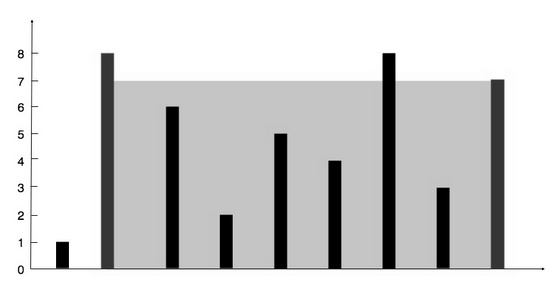# Container With Most Water in Python

Suppose we have a set of n non-negative integers a1, a2, ..., an,each value represents a point at coordinate (i, a[i]). n vertical lines are present in such a way that the two endpoints of line i is at (i, a[i]) and (i, a). We have to find two lines, which together with x-axis forms one container, so our goal is to find two columns where water volume is max. So if the array is like [1,8,6,2,5,4,8,3,7], then it will beIn the shaded part, the height is 7 and there are 7 sections, so the total area is actually 7 * 7 = 49. This is the output.

To solve this, we will follow these steps

• low := 0, high := length of arr – 1, ans := 0
• while low < high
• if arr[low] < arr[high]: min_h := height[low] and min_ind := low
• otherwise min_h := height[high] and min_ind := high
• ans := maximum of (high – low)* min_h and ans
• if low + 1 = min_ind + 1, then increase low by 1 otherwise decrease high by 1
• return ans

## Example

Let us see the following implementation to get better understanding −

Live Demo

class Solution(object):
def maxArea(self, height):
low = 0
high = len(height) - 1
ans = 0
while low < high:
if height[low]<height[high]:
min_height = height[low]
min_height_index = low
else:
min_height = height[high]
min_height_index = high
ans = max(((high - low) ) * min_height,ans)
if low+1==min_height_index+1:
low+=1
else:
high-=1
return ans
ob1 = Solution()
print(ob1.maxArea([1,8,6,2,5,4,8,3,7]))

## Input

[1,8,6,2,5,4,8,3,7]

## Output

49